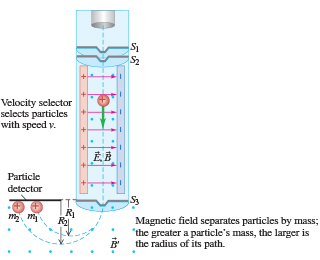# Problem: The amount of meat in prehistoric diets can be determined by measuring the ratio of the isotopes nitrogen-15 to nitrogen-14 in bone from human remains. Carnivores concentrate 15N, so this ratio tells archaeologists how much meat was consumed by ancient people. Suppose you use a velocity selector (Figure 1) to obtain singly ionized (missing one electron) atoms of speed 513 km/s and want to bend them within a uniform magnetic field of 0.510 T. The measured masses of these isotopes are 2.35×10-26 kg (14N) and 2.45×10-26 kg (15N).Find the separation of the 14N and 15N isotopes at the detector.Express your answer with the appropriate units.s = ___

###### FREE Expert Solution

Magnetic force must be equal to the centripetal force.

Therefore,

$\overline{)\frac{\mathbf{m}{\mathbf{v}}^{\mathbf{2}}}{\mathbf{r}}{\mathbf{=}}{\mathbf{q}}{\mathbf{v}}{\mathbf{B}}\phantom{\rule{0ex}{0ex}}\frac{\mathbf{m}\mathbf{v}}{\mathbf{r}}{\mathbf{=}}{\mathbf{q}}{\mathbf{B}}}$

Solving for r:

$\overline{){\mathbf{r}}{\mathbf{=}}\frac{\mathbf{m}\mathbf{v}}{\mathbf{q}\mathbf{B}}}$

For N - 14,

rN-14 = (2.35×10-26)(513×103)/(1.6 × 10-19)(0.510) = 0.1477 m

Distance, d14 = 2r14 = (2)(0.1477) = 0.2954  m

88% (484 ratings)###### Problem Details

The amount of meat in prehistoric diets can be determined by measuring the ratio of the isotopes nitrogen-15 to nitrogen-14 in bone from human remains. Carnivores concentrate 15N, so this ratio tells archaeologists how much meat was consumed by ancient people. Suppose you use a velocity selector (Figure 1) to obtain singly ionized (missing one electron) atoms of speed 513 km/s and want to bend them within a uniform magnetic field of 0.510 T. The measured masses of these isotopes are 2.35×10-26 kg (14N) and 2.45×10-26 kg (15N).Find the separation of the 14N and 15N isotopes at the detector.